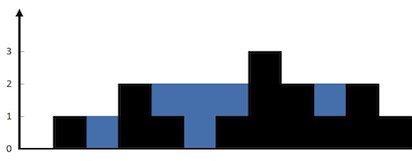## 引例 - 装水容器

• 忽略墙壁的厚度
• 忽略选定的墙壁之间的墙壁### 暴力解法

  1 2 3 4 5 6 7 8 9 10 11  class Solution { fun maxArea(height: IntArray): Int { var maxArea = 0 for (a in height.indices) { for (b in a + 1 until height.size) { maxArea = max(maxArea, min(height[a], height[b]) * (b - a)) } } return maxArea } } 

### 优化

4, 8, 1, 2, 9
p1          p2


1. p1 后移。那么有两种可能的结果：
1. 新的数 >4，可能得到更大的容积 ✅
2. 新的数 <=4，只会得到更小的容积 ❌
2. p2 前移。也是两种情况：
1. 新的数 >4，但只会得到更小的容积 ❌ 因为第一个因数还是取 p1=4 不变，宽度却变小了。
2. 新的数 <=4，显然，容积更小了 ❌

a=b 的边界情况无需特殊处理，随便选一个移动就好。此时容积一定会减小，但不能由此认为算法应该终止，因为后面依然有变大的可能。

### 优化后代码

  1 2 3 4 5 6 7 8 9 10 11 12 13 14 15 16  class Solution { fun maxArea(height: IntArray): Int { // 只有移动小的一边，结果才有可能变大。 var maxArea = 0 var a = 0 var b = height.size - 1 while (a < b) { maxArea = max(min(height[a], height[b]) * (b - a), maxArea) if (height[a] < height[b]) a++ else b-- } return maxArea } } 

## 正餐 - 收集雨水

LeetCode42 - Trapping Rain Water

• 墙壁厚度不可忽略
• 墙壁会占用容积### 暴力解法

  1 2 3 4 5 6 7 8 9 10 11 12 13 14 15 16 17 18 19 20 21 22 23 24  class Solution { fun trap(height: IntArray): Int { // 单独计算每列的容积 v = min(maxL,maxR)-h var total = 0 for (i in height.indices) { val v = maxBoundary(height, i) - height[i] if (v > 0) total += v } return total } private fun maxBoundary(height: IntArray, pos: Int): Int { var maxL = 0 for (i in 0 until pos) { maxL = max(maxL, height[i]) } var maxR = 0 for (i in pos + 1 until height.size) { maxR = max(maxR, height[i]) } return min(maxL, maxR) } } 

### 优化

0, 1, 0, 2, 1, 0, 1, 3, 2, 1, 2, 1
p1                               p2


0, 1, 0, 2, 1, 0, 1, 3, 2, 1, 2, 1
p1                         p2


1. 对较矮的一边进行操作。
2. 若高度低于同侧的最大高度则计算容积；
3. 否则更新最大高度。
4. 向中间移动指针，

### 优化后代码

  1 2 3 4 5 6 7 8 9 10 11 12 13 14 15 16 17 18 19 20 21 22 23 24 25 26 27  class Solution { fun trap(height: IntArray): Int { var a = 0 var b = height.size - 1 var maxL = 0 var maxR = 0 var total = 0 while (a < b) { if (height[a] < height[b]) { if (height[a] > maxL) { maxL = height[a] } else { total += maxL - height[a] } a++ } else { if (height[b] > maxR) { maxR = height[b] } else { total += maxR - height[b] } b-- } } return total } }